# NCERT Solutions: Light Notes | Study NCERT Hindi Textbooks (Class 6 to Class 12) - UPSC

## UPSC: NCERT Solutions: Light Notes | Study NCERT Hindi Textbooks (Class 6 to Class 12) - UPSC

The document NCERT Solutions: Light Notes | Study NCERT Hindi Textbooks (Class 6 to Class 12) - UPSC is a part of the UPSC Course NCERT Hindi Textbooks (Class 6 to Class 12).
All you need of UPSC at this link: UPSC

Q1. Fill in the blanks:
(a) An image that cannot be obtained on a screen is called __.
(b) Image formed by a convex ____ is always virtual and smaller in size.
(c) An image formed by a ____ mirror is always of the same size as that of the object.
(d) An image which can be obtained on a screen is called a ___image.
(e) An image formed by a concave ___cannot be obtained on a screen.
Ans:

(a) An image that cannot be obtained on a screen is called virtual image.
Reason: A virtual image cannot be obtained on a screen. Only real images can be obtained on a screen.
(b) Image formed by a convex mirror is always virtual and smaller in size.
Reason: A convex mirror forms a virtual, diminished and upright image of an object.
(c) An image formed by a plane mirror is always of the same size as that of the object.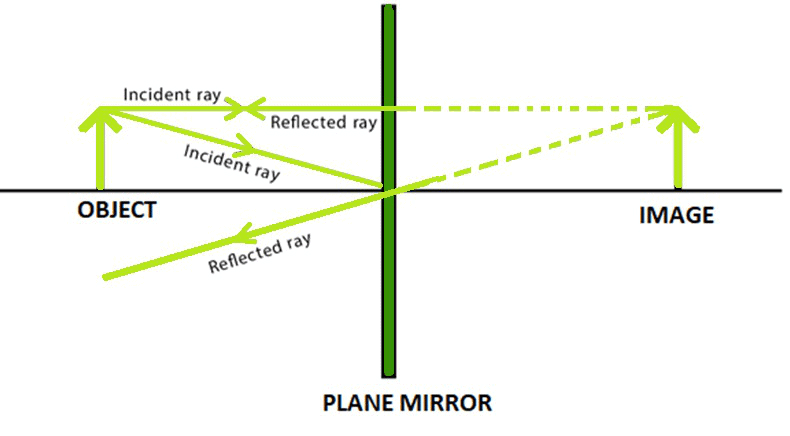(d) An image which can be obtained on a screen is called a real image.
Reason: Only real images can be obtained on a screen.
(e) An image formed by a concave lens cannot be obtained on a screen.
Reason: The image formed by concave lens is always virtual. A virtual image cannot be obtained on a screen.

Q2. Mark ‘T’ if the statement is true and ‘F’ if it is false:
(a) We can obtain an enlarged and erect image by a convex mirror. (T/F)
(b) A concave lens always forms a virtual image. (T/F)
(c) We can obtain a real, enlarged and inverted image by a concave mirror. (T/F)
(d) A real image cannot be obtained on a screen. (T/F)
(e) A concave mirror always forms a real image. (T/F)

Ans:
(a) False, The image formed by a convex mirror is always virtual, diminished and erect.
(b) True, The image formed by a concave lens is always virtual, erect and diminished.
(c) True, The image formed by a concave mirror can be real or virtual, enlarged or diminished, and erect or inverted, depending upon the distance of the object from the mirror.
(d) False, The image that can be obtained on a screen is called a real image. Virtual images cannot be obtained on a screen.
(e) False, A concave mirror can form both real and virtual images. The nature of the image depends on the distance of the object from the mirror.

Q3. Match the items given in Column I with one or more items of Column II.

 Column I Column II (a) A plane mirror (i) Used as a magnifying glass. (b) A convex mirror (ii) Can form image of objects spread over a large area. (c) A convex lens (iii) Used by dentists to see enlarged image of teeth. (d) A concave mirror (iv) The image is always inverted and magnified. (e) A concave lens (v) The image is erect and of the same size as the object. (vi) The image is erect and smaller in size than the object.

Ans:
(a) - (v)
A plane mirror forms an image which is erect and of the same size as the object.

(b) - (ii) and (vi)
A convex mirror forms a virtual, diminished, and upright image and therefore, covers a larger area of view. Its field of view is very large.

(c) - (i)
A convex lens can form a magnified image of the object. Therefore, it is used as a magnifying glass.

(d) - (iii)
A concave mirror forms an enlarged image of the object. Therefore, it is used to see an enlarged image of teeth by the dentist.

(e) - (vi)
The image formed by a concave lens is always virtual, upright and erect.

Q4. State the characteristics of the image formed by a plane mirror.
Ans: The image formed by a plane mirror is erect, laterally inverted, and of the same size as that of the object. The left side of the object appears on the right side of the image. Also, the distance of the image from the plane mirror is same as the distance between the plane mirror and the object.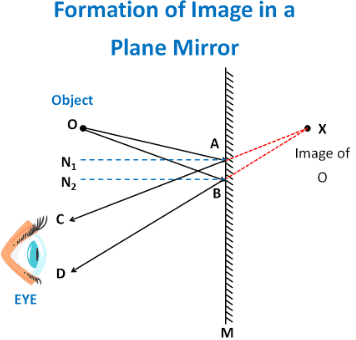Q5. Find out the letters of English alphabet or any other language known to you in which the image formed in a plane mirror appears exactly like the letter itself. Discuss your findings.
Ans: If the letters of English alphabet A, H, I, M, O, T, U, V, W, X, Y are kept in front of a plane mirror, then they would form images which exactly look like the original letters of the alphabet. These letters are vertically symmetric. For example, if we divide letters A and U in the middle, then we would find that the right halves are equivalent to the left halves of the letters.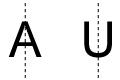Hence, even if the image interchanges sidewise, it will appear same as the letter.

Q6. What is a virtual image? Give one situation where a virtual image is formed.
Ans: When an image cannot be obtained on a screen, it is called a virtual image.
The image formed by a plane mirror is virtual, because the image cannot be obtained on a screen when placed either in front of the mirror or behind it.

Q7. State two differences between a convex and a concave lens.
Ans:
(i) Convex lens is thicker in the middle while concave lens is thinner in the middle.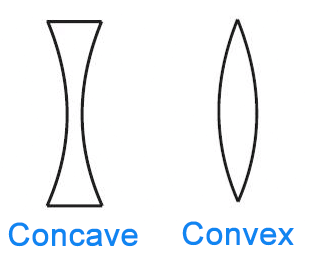(ii) A convex lens converges the light ray falling on it, while a concave lens diverges the light ray falling on it.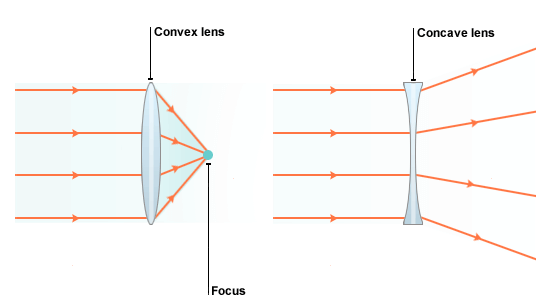Q8. Give one use each of a concave and a convex mirror.
Ans: Concave mirrors can form enlarged image of the object. Therefore, they are used by the dentist to see the enlarged image of the patient’s teeth.
Convex mirror forms diminished and upright image of the object. It is used as a side view mirror of the car because it enables the driver to view objects spread over a large area behind him/her.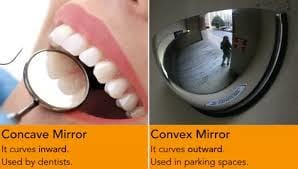Q9. Which type of mirror can form a real image?
Ans: A concave mirror can form real image of an object. The nature of the image depends on the distance of the object from the concave mirror.

Q10. Which type of lens forms always a virtual image?
Ans: A concave lens always forms a virtual image of an object.

Q11. A virtual image larger than the object can be produced by a
(i) Concave lens
(ii) Concave mirror
(iii) Convex mirror
(iv) Plane mirror

Ans: (ii) Concave mirror
Concave mirror can form virtual and magnified image of the object.
Concave lens and convex mirror forms virtual but diminished image of the object. Plane mirror forms virtual and same size image of the object.

Q12. David is observing his image in a plane mirror. The distance between the mirror and his image is 4 m. If he moves 1 m towards the mirror, then the distance between David and his image will be
(i) 3 m
(ii) 5 m
(iii) 6 m
(iv) 8 m

Ans: (iii) 6 m
In the case of a plane mirror, the distance between the object and the mirror (d1) is same as the distance between the image and the mirror (d2).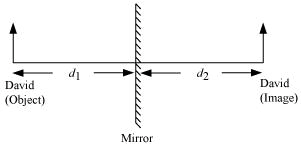Given,
Distance between the mirror and David’s image, d2 = 4 m
Therefore, d1 = d2 = 4 m
If David moves 1 m towards the mirror, then d1 = 4 − 1 = 3 m
Again, d1 = d2 = 3 m
Therefore, the distance between David and his image is d1 + d2 = 3 + 3 = 6 m.

Q13. The rear view mirror of a car is a plane mirror. A driver is reversing his car at a speed of 2 m/s. The driver sees in his rear view mirror the image of truck parked behind his car. The speed at which the image of the truck appears to approach the driver will be:
(i) 1 m/s
(ii) 2 m/s
(iii) 4 m/s
(iv) 8 m/s

Ans: (iii) 4 m/s
The speed of the car is 2 m/s which means the car is approaching the truck with a speed of 2 m per second. The distance between the car and truck will decrease at a double rate. This is because the image of the truck will travel a distance twice the distance travelled by the car in equal time. Hence, the image of the truck will appear to approach the driver with the speed of 2 ×2 = 4 m/s.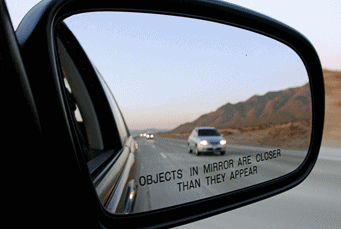Rear View Mirror

The document NCERT Solutions: Light Notes | Study NCERT Hindi Textbooks (Class 6 to Class 12) - UPSC is a part of the UPSC Course NCERT Hindi Textbooks (Class 6 to Class 12).
All you need of UPSC at this link: UPSCUse Code STAYHOME200 and get INR 200 additional OFF

## NCERT Hindi Textbooks (Class 6 to Class 12)

974 docs|404 tests

### How to Prepare for UPSC

Read our guide to prepare for UPSC which is created by Toppers & the best Teachers

Track your progress, build streaks, highlight & save important lessons and more!

,

,

,

,

,

,

,

,

,

,

,

,

,

,

,

,

,

,

,

,

,

;